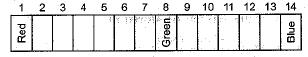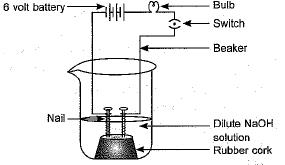Courses

# Acids And Bases-2

## 20 Questions MCQ Test Science Class 10 | Acids And Bases-2

Description
This mock test of Acids And Bases-2 for Class 10 helps you for every Class 10 entrance exam. This contains 20 Multiple Choice Questions for Class 10 Acids And Bases-2 (mcq) to study with solutions a complete question bank. The solved questions answers in this Acids And Bases-2 quiz give you a good mix of easy questions and tough questions. Class 10 students definitely take this Acids And Bases-2 exercise for a better result in the exam. You can find other Acids And Bases-2 extra questions, long questions & short questions for Class 10 on EduRev as well by searching above.
QUESTION: 1

### Which of the following gives the correct increasing order of acidic strength ?

Solution:

Hydrochloric acid is a strong acid (inorganic), acetic acid is a weak acid (organic).

QUESTION: 2

### Which of the following statements is true for acids?

Solution:

Acids are sour in taste and turns blue litmus to red

QUESTION: 3

### Calcium phosphate is present in tooth enamel. Its nature is

Solution:

Calcium phosphate Ca3(PO4)2  is a salt of strong base Ca(OH)2 and weak acid, phosphoric acid.
Therefore, it is basic.

QUESTION: 4

Which of the following is(are) true when HCI (g) is passed through water ?
(i) It does not ionise in the solution as it is a covalent compound.
(ii) It ionises in the solution.
(iii) It gives both hydrogen and hydroxyl ion in the solution.
(iv) It forms hydronium ion in the solution due to the combination of hydrogen ion with water molecule.

Solution:

HCl + H2O →H3O+ + Cl-

QUESTION: 5

Which one of the following can be used as an acid-base indicator by a visually impared student ?

Solution:

Vanilla essence makes use of smell. Therefore, it can be used by a visually impaired student. It is a type of olfactory indicator.

QUESTION: 6

Equal volumes of hydrochloric acid and sodium hydroxide solutions of same concentration are mixed and the pH of the resulting solution is checked with a pH paper. What would be the colour obtained ? (You may use colour guide given in figure)Solution:

Hcl being strong acid and NaOH being strong base reacts to form a neutral salt which gives green color. It is a neutralization reaction.

QUESTION: 7

Common salt besides being used in kitchen can also be used as the raw material for making
(i) washing soda
(ii) bleaching powder
(iii) baking soda
(iv) slaked lime

Solution:

A. Baking soda is sodium hydrogen carbonate which is prepared by Solvay process which is the reaction of sodium chloride,ammonia and carbon dioxide in water. The calcium carbonate is used in the reaction to get carbon dioxide gas.
CaCO33→ CaO + CO

2NH3+ CO​+ H2​O → (NH4​)2​CO​+ CO​+ H2​O → NH4​HCO3

​ NH4​HCO+ NaCl → NaHCO​+ NH4​Cl
B. Washing soda is sodium carbonate which is prepared from the sodium chloride.
2NaCl + CaCO​→ Na2​CO​+ CaCl2

QUESTION: 8

Which of the following statement is not correct?

Solution:

Metal oxide react with water to form bases but salt and acid is not formed.
NaO + H2O → NaOH + H2

QUESTION: 9

Sodium carbonate is a basic salt because it is a salt of

Solution:

Salts of weak acid and strong base behave as basic salts.
H2CO3 + 2NaOH → Na2CO3 + 2H2O

QUESTION: 10

Which among the following is not a base ?

Solution:

C2H5OH (ethyl alcohol) does not dissociate into OH- ions on dissolving in water.

QUESTION: 11

Which of the following statements is correct about an aqueous solution of an acid and of a base ?
(i) Higher the pH, stronger the acid
(ii) Higher the pH, weaker the acid
(iii) Lower the pH, stronger the base
(iv) Lower the pH, weaker the base

Solution:

Acidic solutions have pH less than 7 and basic solutions have pH more than 7

QUESTION: 12

Which of the following is used for dissolution of gold ?

Solution:

Aqua - regia also known as royal water is a mixture of (3 part HCl & 1 part HNO3 ) which dissolves even noble metals like Au, Pt.

QUESTION: 13

Which of the following salts does not contain water of crystallisation ?

Solution:

Blue vitriol is CuS04.5H20, washing soda is Na2CO3.10H2O and gypsum is CaSO4.2H2O. Baking soda does not contain water of crystallisation it is NaHCO3.

QUESTION: 14

In an attempt to demonstrate electrical conductivity through an electrolyte, the following apparatus was set up.Which among the following statement(s) is (are) correct?

(i) Bulb will not glow because electrolyte is not acidic.
(ii) Bulb will glow because NaOH is a strong base: and furnishes ions for conduction.
(iii) Bulb will not glow because circuit is incomplete.
(iv) Bulb will not glow because it depends upon the type of electrolytic solution.

Solution:

NaOH being a strong electrolyte conducts electricity in aqueous solution. Moreover the circuit is also complete.

QUESTION: 15

During the preparation of hydrogen chloride gas on a humid day, the gas is usually passed through the guard tube containing calcium chloride. The role of calcium chloride taken in the guard tube is to

Solution:

Calcium chloride has the property of absorbing water vapours

QUESTION: 16

Which one of the following can be used as an acid-base indicator by a visually impared (blind) Student ?

Solution:
QUESTION: 17

What happens when a solution of an acid is mixed with a solution of a base in a test tube ?
(i) The temperature of the solution increases.
(ii) The temperature of the solution decreases.
(iii) The temperature of the solution remains the same.
(iv) Salt formation takes place.

Solution:

Mixing of an acid and a base is an exothermic process and is accompanied by the formation of a salt Neutralization reaction.

QUESTION: 18

Which of the following phenomena will occur, when a small amount of acid is added to water?
(i) Ionisation
(ii) Neutralisation
(iii) Dilution
(iv) Salt formation

Solution:

When acid is added to water it ionises into H+ ion and concentration of acid decreases i.e. Dilution

QUESTION: 19

A sample of soil is mixed with water and allowed to settle. The clear supernatant solution turns the pH paper yellowish-orange. Which of the following would change the colour of this pH paper to greenish-blue?

Solution:

Antacid is basic in nature and turns the pH paper to blue color.

QUESTION: 20

An aqueous solution turns red litmus solution blue. Excess addition of which of the following solution would reverse the change?

Solution:

Baking powder is sodium bicarbonate. Lime is calcium oxide or hydroxide. Ammonium hydroxide, lime and baking powder all three are basic in nature. The have the property of changing red litmus to blue while HCl is hydrochloric acid which can turn blue litmus to red as this is a very strong acid.